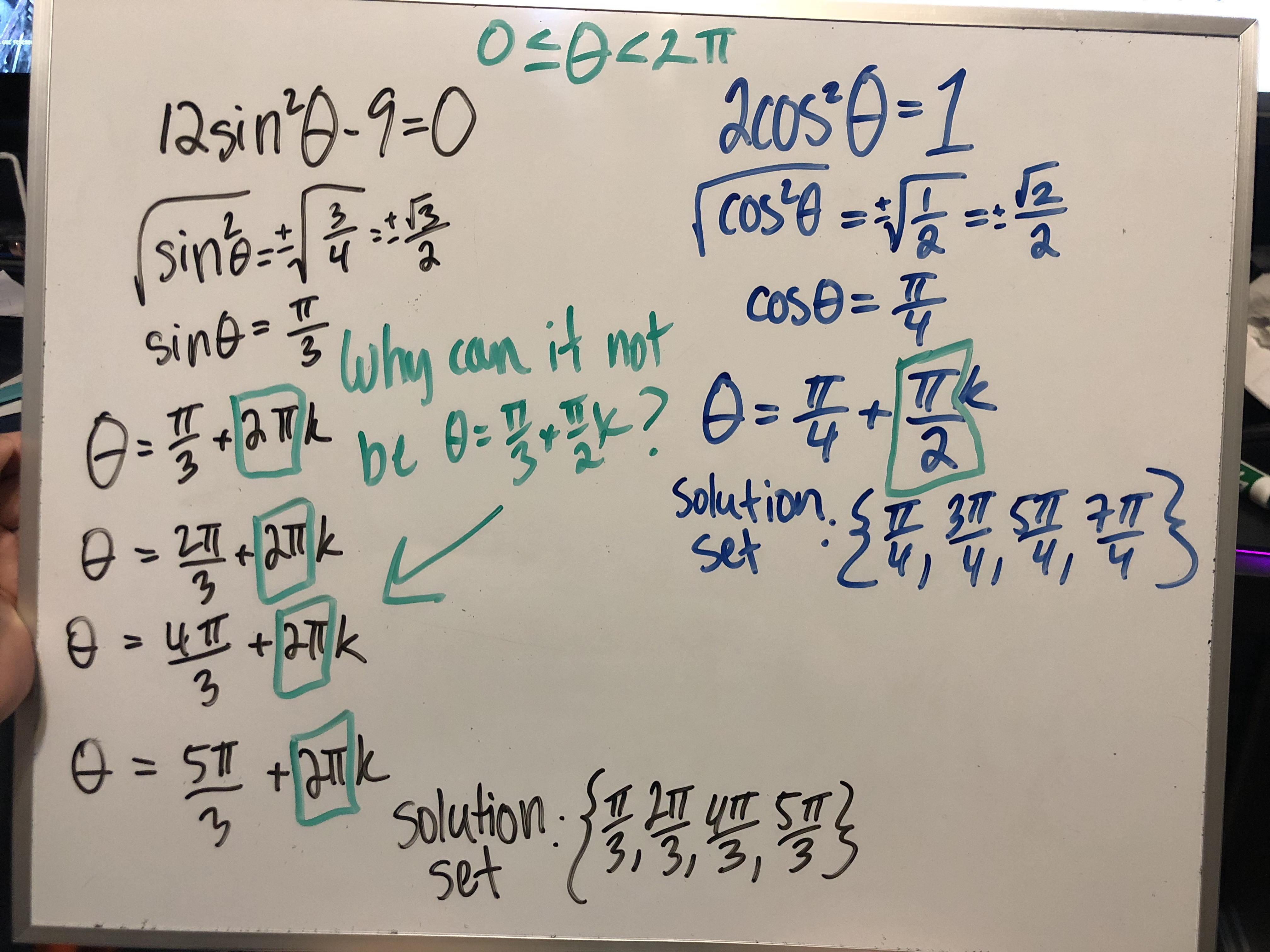# 12sin'-9-0Jcos-1COS=cosesinewhy can it notCOSE=SolutionSet3t S4T3=51 +kSolutionSet.t

Question

Hello,

I am having trouble in finding the difference between these equations to find the solutions. 2pi vs. pi/2help_outlineImage Transcriptionclose12sin'-9-0 Jcos-1 COS= cose sinewhy can it not COSE= Solution Set 3 t S 4T 3 =51 +k Solution Set .t fullscreen
check_circle

star
star
star
star
star
1 Rating
Step 1

Consider the provided trigonometry equation.

Step 2

Now the above equation can be written as:

Step 3

As we know in first and second quadrant the value of sin (θ) is ...

### Want to see the full answer?

See Solution

#### Want to see this answer and more?

Solutions are written by subject experts who are available 24/7. Questions are typically answered within 1 hour.*

See Solution
*Response times may vary by subject and question.
Tagged in

### Calculus